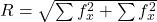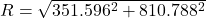## There are 3 people pulling on an object, the first person pulls with a force of 226 newtons at a direction of 145 degrees the second person

Question

There are 3 people pulling on an object, the first person pulls with a force of 226 newtons at a direction of 145 degrees the second person pulls with a force of 331 newtons at a direction of 303 degrees. The third person pulls with a force of 377 newtons at a direction of 77 degrees. find the resultant vector . find the resultant force . find the direction

in progress 0
2 months 2021-07-18T23:33:43+00:00 1 Answers 1 views 0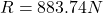Step-by-step explanation:

From the question we are told that:

Force 1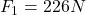Force 2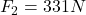Force 3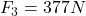Direction 1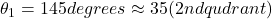Direction 2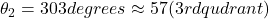Direction 3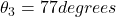Generally Resolving forces to X axis is mathematically given by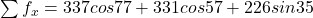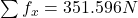Generally Resolving forces to Y axis is mathematically given by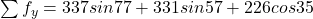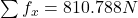Generally the equation for Resultant force R is mathematically given by